# Mathematics - Signals And Systems - Exercises on Filtering

in StemSocial6 months ago[Image1]

## Introduction

Hey it's a me again @drifter1!

Today we continue with my mathematics series about Signals and Systems in order to cover Exercises on Filtering.

So, without further ado, let's dive straight into it!

## Filtering Recap

Filtering is based upon the convolution property of the Fourier Transform: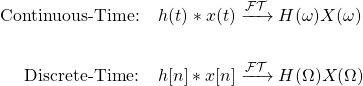The convolution property of the Fourier Transform allows us to simplify the calculations for LTI Systems (by moving into the Frequency-Domain), but it can also be used as a way of "re-shaping" the input. Depending on the frequency bands which are "allowed" to pass, filters are categorized into low-pass, high-pass and band-pass. An approximation of the ideal filters can be implemented using series R-C circuits and moving average filters.

## Filter Properties [Based on 12.1 from Ref1]

Consider a filter with frequency response H(ω) as shown in the following Figure: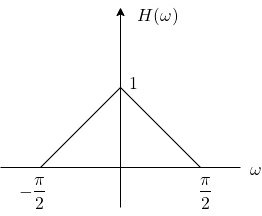Check which of the following properties the filter satisfies:

• Real-valued or Complex-valued
• Even or Odd
• Causal or Non-Causal

### Solution

First of all the impulse response is real because: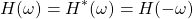and so: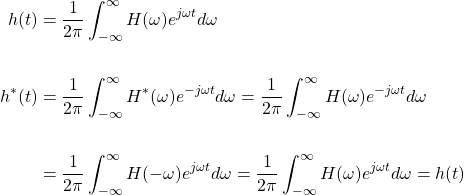The graph of the impulse response reflects across the y-axis, which means that the function is even. Mathematically: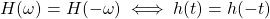Last but not least, the impulse response is also non-causal because: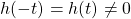## Lowpass, Highpass or Bandpass Filter [Based on 12.5 from Ref1]

Determine the type of filter the following filters correspond to: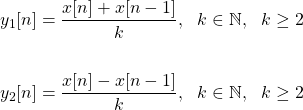### Solution

Examining the mathematical expressions of those filters its easy to notice that y1[n] supresses changes by averaging x[n], whilst y2[n] supresses the frequency bands that have varied (from x[n-1] to x[n]). As such, y1[n] can be thought of as an low-pass filter, whilst y2[n] can be used as a high-pass filter. As the value of k increases we suspect that the changes will be supressed further.

The Fourier Transforms of these two simple cases can be calculated using properties, which easily gives us the following frequency responses: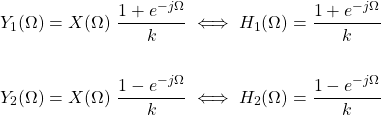For k = 2, and in the range [-2π, 2π] the first filter closes up to zero at +-π, whilst the second filter has a value of zero at 0, +-2π. As such, the first filter is closer to a low-pass filter, whilst the second filter is closer to a high-pass filter.

## Filter Output [Based on 12.8 from Ref1]

Consider a filter with the following impulse response: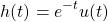Calculate the frequency response H(Ω) and determine the filter output for the following input signals: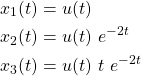### Solution

The impulse response is one of the known FT pairs, and as such the frequency response is: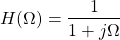The Fourier Transforms of the input signals are also in FT pair tables and as such: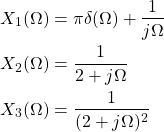The Filter output for each of these cases is: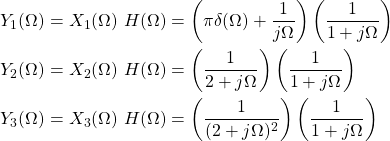Getting back to the Time Domain might be quite difficult for the first case, so let's only do this for the second case (which we've done a handful of times) and I will leave the third output as an exercise for the reader. :)

We first have to turn the multiplication into a sum using factor simplification, which gives us the following: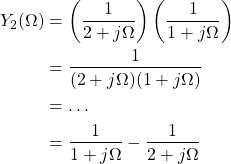The final result is a sum of two known FT pairs, and so the output is: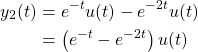## RESOURCES:

### Images

Block diagrams and other visualizations were made using draw.io and GeoGebra

## Final words | Next up

And this is actually it for today's post!

Next time we will get into Modulation examples...

See Ya!Keep on drifting!